Click to Chat

1800-1023-196

+91-120-4616500

CART 0

• 0

MY CART (5)

Use Coupon: CART20 and get 20% off on all online Study Material

ITEM
DETAILS
MRP
DISCOUNT
FINAL PRICE
Total Price: Rs.

There are no items in this cart.
Continue Shopping```Special Matrices

Symmetric and Skew MatricesA square matrix A = [aij] is said to be symmetric when aij = aij for all i and j. If aij = -aij for all i and j and all the leading diagonal elements are zero, then the matrix is called a skew symmetric matrix.

For example: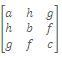is a symmetric matrix and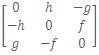is a skew-symmetric matrix.

Hermitian and Skew - Hermitian Matrices

A square matrix A = [aij] is said to be Hermitian matrix if aij =ij,i, j i.e. Aθ = -A.

For example:are Hermitian matrices.

Note:
* If A is a Hermitian matrix then aii =ij is a reali. Thus every   diagonal element of a Hermitian Matrix must be real.
* A Hermitian matrix over the set of real numbers is actually a real symmetric matrix.

And a square matrix, A = [aij] is said to be a skew-Hermitian if aij =
-ij,i, j i.e. Aθ = -A.

For example:are skew-Hermitian matrices.

Note:
* If A is a skew-Hermitian matrix then aii =ij => aii +ii = 0, i.e. aii must be purely imaginary or zero.
* A skew-Hermitian Matrix over the set of real numbers is actually a real skew- symmetric matrix.

Singular and Non-singular Matrices

Any square matrix A is said to be non-singular if |A| ≠ 0, and a square matrix A is said to be singular if |A| = 0. Here |A| (or det(A) or simply det A) means corresponding determinants of square matrix A e.g. if10 - 12 = -2 => A is a non-singular matrix.

Unitary Matrix

A square matrix is said to be unitary if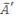A = I. Since || = |A| and |A|=||, we have |||A| = 1.

Thus the determinant of a unitary matrix is of unit modulus. For a matrix to be unitary it must be non-singular.

Hence,A = I => A= I.

Orthogonal Matrix

Any square matrix A of order n is said to be orthogonal if AA' = A'A = In.

Idempotent Matrix

A square matrix A is called idempotent provided it satisfies the relation A2 = A.

For example:

The matrix A =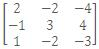is idempotent as

A2 = A.A == A.

Involuntary Matrix

A matrix such that A2 = I is called involuntary matrix.

Nilpotent Matrix

A square matrix A is called a nilpotent matrix if there exists a positive integer m such that Am = O. If m is the least positive integer such that  Am = O, then m is called the index of the nilpotent matrix A

Illustration:

Suppose a, b, c are real numbers such that abc = 1. If A =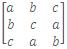is such that A'A = I, then find the value of a3 + b3 + c3.

Solution:

Note A' = A.

Thus, I = A'A = AA = A2

|A2| = |A2| = |I| = 1

|A| = + 1. But |A| = a3 + b3 + c3 - 3abc.

Thus, a3 + b3 + c3 - 3abc = + 1

=> a3 + b3 + c3 = 4, 2.

Illustration:

If ω ≠ 1 is a cube root of unity, then show that

A =is singular matrix.

Solution: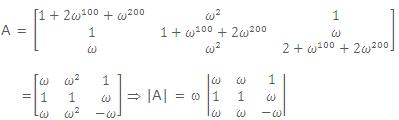Hence A is singular matrix.

Illustration:

Show that the matrix =is nilpotent matrix of index 3.

Solution: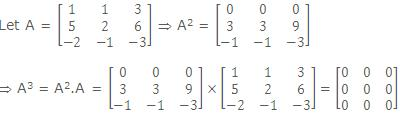=> A3 = 0 i.e. Ak = 0. Here k = 3.

Hence A is nilpotent matrix of index 3.

Let A = [aij] be a square matrix of order n and let Cij be cofactor of aij in A. Then the transpose of the matrix of cofactors of elements of A is called the adjoint of A and is denoted by adj A.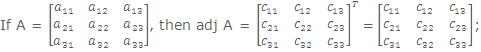where Cij denotes the cofactor of aij in A.

For example:  A =, C11 = s, C12 = -r, C21 = -q, C22 = p

=> adj A =.

Theorem:  Let A be a square matrix of order n. Then A(adj A) = |A| In = (adj A)A.

Proof:      Let A = [aij], and let Cij be cofactor of aij in A. Then

(adj A)ij = Cij1 < i, j , n.

Now, (A(adj A))ij = ∑nr=1(A)ir  (adj A)rj = ∑nr=1 aij Cjr=> A (adj A) == |A| In.

Similarly ((adj A)A)ij= ∑nr=1(adj A)ir (A)rj= ∑nr=1Cri arj=Note :  The adjoint of a square matrix of order 2 can be easily obtained by interchanging the diagonal elements and changing the signs of off-diagonal (left hand side lower corner to right hand side upper corner) elements.

Inverse of a Matrix

A non-singular square matrix of order n is invertible if there exists a square matrix B of the same order such that AB = In = BA.

In such a case, we say that the inverse of A is B and we write, A-1 = B.

The inverse of A is given by A-1 = 1/|A|. adj A.

Properties of Inverse of a Matrix

(i) Every invertible matrix possesses a unique inverse.

(ii) (Reversal law) If A and B are invertible matrices of the same order, then AB is invertible and (AB)-1 = B-1 A-1.

In general,if A,B,C,...are invertible matrices then (ABC....)-1 =..... C-1 B-1 A-1.

(iii) If A is an invertible square matrix, then AT is also invertible and (AT)-1 = (A-1)T.

(iv) If A is a non-singular square matrix of order n, then |adj A| = |A|n-1.

(v) If A and B are non-singular square matrices of the same order, then adj (AB) = (adj B) (adj A).

(vi) If A is an invertible square matrix, then adj(AT) = (adj A)T.

(vii) If A is a non-singular square matrix, then adj(adjA) = |A|n-1A.

To read more, Buy study materials of Matrices and Determinants comprising study notes, revision notes, video lectures, previous year solved questions etc. Also browse for more study materials on Mathematics here.
```### Course Features

• 731 Video Lectures
• Revision Notes
• Previous Year Papers
• Mind Map
• Study Planner
• NCERT Solutions
• Discussion Forum
• Test paper with Video Solution